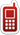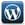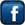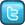MLK Home & Online Tutor+91-836-801-8076+91-999-034-6833Home Tutors available in Ghaziabad, Noida & East Delhi | Ghaziabad- Indirapuram, Vaishali, Vasundhara, Kaushambhi, Shahibabad, Shalimar Garden, Siddharth Vihar, Pratap Vihar, Crossing Republik, Mohan Nagar, Kavi Nagar, Raj Nagar, Shastri Nagar, Nehru Nagar, Patel Nagar, Model Town, Govind Puram, Chiranjeev Vihar, Gandhi Nagar, Lal Kuan, Wave City, Raj Nagar Extension | Noida- Noida(All Sectors), Greater Noida, Noida West(Noida Extension-Gour City-1,2; Amarpali Dream Valley; SuperTech Eco Village) | Delhi- East Delhi(Preet Vihar, Vivek Vihar, Shahdara, Mayur Vihar, Patparganj, Lakshmi Nagar, Shakarpur), South Delhi, West Delhi, North Delhi & Central Delhi.

## AREA WISE CHEMISTRY TUTORSFollow @tutormlk# Math Tutoring for Grade 6- Find Math home Tutors, Online Tutors, home Tuition & Private Tutors for Class VI

Is your child struggling in school? Does your child stuck on math homework?

Or

Are you having difficulty working out math problems? Are you looking out for a grade 6 math home tutor?

MLK Home & Online Tutor can help you find the most qualified home & Online Math tutors for class/grade 6 across Ghaziabad, Noida, Delhi, Bangalore in Karnataka, Pune in Maharashtra, Hyderabad in Andhra Pradesh, Mumbai in Maharashtra, New Delhi in Delhi, Gurgaon in Haryana, Chandigarh in Punjab and Haryana, Noida in Uttar Pradesh, Ahmedabad in Gujarat & Chennai in Tamilnadu.

MLK Home & Online Tutor can help you find Maths tutors for class/grade VI we have available near you. We will send him to your home for one free trial class, if satisfied with his tutoring, then continue. We are the only place, where just a single Call or Message will recommend you Maths tutor class/grade 6 for your personal needs, arranged according to their rates and distance from you. Maths can be an extremely tough subject to get to grips with, but private Maths tuition can really help your learning!

If you are a class/grade VI Maths tutor wishing to provide home tutoring in Maths class/grade 6, or in any of our other subjects, please register with MLK Home & Online Tutor.

Click here for a Home Tutor or an Online Tutor? MLK Home & Online Tutoring Service will assist your child in simplifying concepts and building confidence in approaching math. We serve students from Grade 6-12. In addition to math, tutoring is available in all core subjects-Physics, Chemistry, General science, Social studies test preparation including OLYNPIADS, NTSE exams, SAT, GMAT, GRE, MAT, CAT and foundation course for AIEEE and IIT-JEE.

## Get the Math Help Now!

Getting help is simple. You just tell us what you are working on and we match a professional Home tutor. Our expert, private, professional home tutor helps you solve your math problems step by step until your home work is finished. Home tutor at MLK Home and Online Tutoring Service provides you with worksheet and problem sets to practice your skills.

## Get a Home Tutor for Math Now!

Our home tutor is trained to identify the exact obstacle that may be hindering students' studies. He supplements the course's curriculum to ensure efficient and motivating home tutoring. Our home tutors are flexible in their schedule as they are available seven days a week for In-home tutoring.

## Affordable Math Test Preperation Programs

MLK Home and Online Tutoring Service 's Math Refresher course is designed to build a strong foundation in the basic math skills essential for success on the following tests:

 SAT GMAT GRE CAT MAT IIT-JEE AIEEE OLYMPIADS NDA SSC Bank PO/Clerk RRB Delhi & UP-SI Exam

## Topics Covered by our Math Home Tutoring Program:

 Arithmetic Pre-Algebra Algebra 1 Algebra 2 Linear Algebra Geometry Pre-Calculus Calculus Statistics & Probability Trigonometry General Math

## Math Sallabus for Grade VI

### Number System

#### (i) Knowing our Numbers:

• Consolidating the sense of numberness up to 5 digits, Size, estimation of numbers, identifying smaller, larger, etc;
• Place value (recapitulation and extension);
• connectives: use of symbols =, <, > and use of brackets;
• word problems on number operations involving large numbers up to a maximum of 5 digits in the answer after all operations. This would include conversions of units of length & mass (from the larger to the smaller units);
• estimation of outcome of number operations;
• Introduction to a sense of the largeness of, and initial familiarity with, large numbers up to 8 digits and approximation of large numbers);

#### (ii) Playing with Numbers:

• Simplification of brackets;
• Multiples and factors;
• divisibility rule of 2, 3, 4, 5, 6, 8, 9, 10, 11;
• Even/odd and prime/composite numbers,Co-prime numbers, prime;
• factorisation, every number can be written as products of prime factors;
• HCF and LCM;
• prime factorization and division method for HCF and LCM;
• the property LCM × HCF = product of two numbers;

#### (iii) Whole numbers

• Natural numbers, whole numbers;
• properties of numbers (commutative, associative, distributive, additive identity, multiplicative identity);
• number line;
• Seeing patterns, identifying and formulating rules to be done by children;

#### (iv) Negative Numbers and Integers

How negative numbers arise, models of negative numbers, connection to daily life, ordering of negative numbers, representation of negative numbers on number line. Children to see patterns, identify and formulate rules. What are integers, identification of integers on the number line, operation of addition and subtraction of integers, showing the operations on the number line (addition of negative integer reduces the value of the number) comparison of integers, ordering of integers.

#### (v) Fractions:

• Revision of what a fraction is
• Fraction as a part of whole
• Representation of fractions (pictorially and on number line)
• fraction as a division, proper, improper & mixed fractions
• equivalent fractions
• comparison of fractions
• addition and subtraction of fractions (Avoid large and complicated unnecessary tasks)
• Review of the idea of a decimal fraction
• place value in the context of decimal fraction
• inter conversion of fractions and decimal fractions (avoid recurring decimals at this stage)
• word problems involving addition and subtraction of decimals (two operations together on money, mass, length and temperature)

### Algebra

#### Introduction to Algebra

• Introduction to variable through patterns and through appropriate word problems and generalizations (example 5 × 1 = 5 etc.);
• Generate such patterns with more examples;
• Introduction to unknowns through examples with simple contexts (single operations);
• ### Ratio and Proportion

• Concept of Ratio;
• Proportion as equality of two ratios;
• Unitary method (with only direct variation implied);
• Word problems;

### Geometry

#### (i) Basic geometrical ideas (2 -D): Introduction to geometry. Its linkage with and reflection in everyday experience

• Line, line segment, ray;
• Open and closed figures;
• Interior and exterior of closed figures;
• Curvilinear and linear boundaries;
• Angle — Vertex, arm, interior and exterior;
• Triangle — vertices, sides, angles, interior and exterior, altitude and median;
• Quadrilateral — Sides, vertices, angles, diagonals, adjacent sides and opposite sides (only convex quadrilateral are to be discussed), interior and exterior of a quadrilateral;
• Circle — Centre, radius, diameter, arc, sector, chord, segment, semicircle, circumference, interior and exterior;

#### (ii) Understanding Elementary Shapes (2-D and 3-D):

• Measure of Line segment;
• Measure of angles
• Pair of lines – Intersecting and perpendicular lines – Parallel lines
• Types of angles- acute, obtuse, right, straight, reflex, complete and zero angle
• Classification of triangles (on the basis of sides, and of angles)
• Types of quadrilaterals – Trapezium, parallelogram, rectangle, square, rhombus
• Simple polygons (introduction) (Upto octagons regulars as well as non regular)
• Identification of 3-D shapes: Cubes, Cuboids, cylinder, sphere, cone, corresponding, interior, exterior angles) prism (triangular), pyramid (triangular and square) Identification and locating in the surroundings
• Elements of 3-D figures. (Faces, Edges and vertices)
• Nets for cube, cuboids, cylinders, cones and tetrahedrons

#### (iii) Symmetry: (reflection)

• Observation and identification of 2-D symmetrical objects for reflection symmetry
• Operation of reflection (taking mirror images) of simple 2-D objects
• Recognising reflection symmetry (identifying axes)

#### (iv) Constructions (using Straight edge Scale, protractor, compasses)

• Drawing of a line segment
• Construction of circle
• Perpendicular bisector
• Construction of angles (using protractor)
• Angle 60°, 120° (Using Compasses)
• Angle bisector- making angles of 30°, 45°, 90° etc. (using compasses)
• Angle equal to a given angle (using compass)
• Drawing a line perpendicular to a given line from a point a) on the line b) outside the line

### Mensuration

#### Concept of Perimeter and Introduction to Area

• Introduction and general understanding of perimeter using many shapes
• Shapes of different kinds with the same perimeter
• Concept of area, Area of a rectangle and a square Counter examples to different misconcepts related to perimeter and area
• Perimeter of a rectangle – and its special case – a square
• Deducing the formula of the perimeter for a rectangle and then a square through pattern and generalisation

### Data handling

• What is data - choosing data to examine a hypothesis?
• Collection and organisation of data - examples of organising it in tally bars and a table
• Pictograph- Need for scaling in pictographs interpretation & construction
• Making bar graphs for given data interpreting bar graphs+Follow @tutormlkFollow @tutormlk